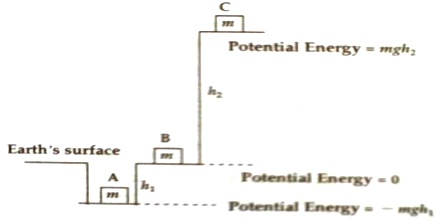Physics

# Can Potential Energy be Negative?Direction: Potential energy can be negative. For example, potential energy at any point above earth’s surface is positive. Potential energy inside a mine is negative [Figure].In this figure potential energy at point B on the earth’s surface is zero. At height h2 at point C potential energy is mgh2. While coming down from point C to B Potential energy starts decreasing. Similarly going from point B to bottom point A potential energy will decrease. Since potential energy at point B is zero, so potential energy at point A will be negative. If A is at depth h, then potential energy at that point will be – mgh1. If the body is to be taken again from point A to B, work is to be done against the weight of the body or against force of gravity. Because a negative amount of work is done to bring an object closer to the Earth, gravitational potential energy is always a negative number when using this reference point. For example, if the datum is defined as the top of a table, and the object is on the floor, that object has a negative potential energy since the height below the top of the table.